# AP Chemistry : Reaction Types

## Example Questions

### Example Question #1 : P H

What is the pH of a 0.05M solution of hydroflouric acid?

3.0

4.5

5.0

7.0

3.0

Explanation:

The Ka = [H+][F–]/[HF]. The beginning concentration of HF is given as 0.05, and we can use x as the variable that accounts for how much of the HF is lost, along with how much of the H+ and F– are formed.

Thus, Ka = x2/.05 if you use the approximation that x is negligible compared to the starting concentration of HF.

Solving for x, x = 1 * 10–3. This is the H+ ion concentration.

pH = –log(H+) = –log(1.0 * 10–3)) = 3

### Example Question #1 : Acid Base Reactions

Which of the following will produce the solution with the lowest pH?

0.2M HCl

0.5M HF

2.0M NaOH

0.3M HI

0.3M HI

Explanation:

NaOH is a base, so that won't produce an acidic solution. Of the remaining acids, HCl and HI are strong acids, and HF is weak. HI is at a higher molarity, so it will produce the most acidic solution.

### Example Question #13 : Reaction Types

A chemist adds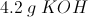to 500 mL of water. What is the pH of the solution?

13.18

0

7.00

0.8248

14.0

13.18

Explanation:

First, we will calculate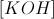as follows: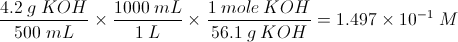Now we will calculate the pOH: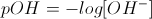In solution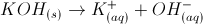, so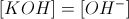.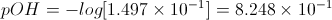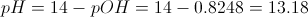### Example Question #63 : Ap Chemistry

A chemist boils off the water from 627 mL of a solution with a pH of 10.2. The only dissolved compound in the solution is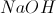. How much solid remains?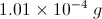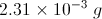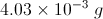No solid remains.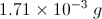Explanation:

The pH of the solution is 10.2, and we know: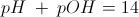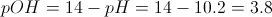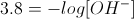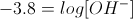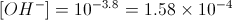And since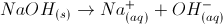,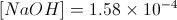.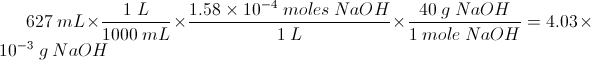### Example Question #11 : P H

A chemist adds 10mL of a solution with a pH of 3.00 to 50mL of water. What is the pH of the new solution?

4.25

3.52

2.22

3.78

3.012

3.78

Explanation:

First, we will determine the amount of hydronium ions in the first solution. This allows us to measure the moles of hydronium ion that are transferred to water.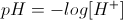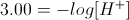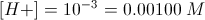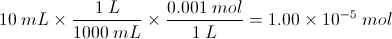Now we can calculate the hydronium ion concentration in the new solution, using the amount transferred and the volume of water, and can solve for pH.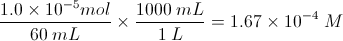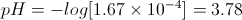### Example Question #11 : P H

Which two solutions, when combined in equal amounts, will produce the lowest pH?

2.0M HCl and 2.0M KOH

1.0M HCl and 1.0M NaCl

2.5M NH3 and 2.5M NH4+

0.5M acetic acid and 1.0M NaCl

1.0M HCl and 1.0M NaCl

Explanation:

HCl is a strong acid, while NaCl is a salt. Mixing these two will result in a pH < 1.

As for the other choices, mixing 2.0M HCl and 2.0M KOH will result in the formation of KCl and H2O, resulting in a neutral pH. Acetic acid (pKa= 4.76) is a weak acid, so it will have a pH less than 7 but greater than 1. Finally, NH3 and NH4+ is a buffer system between a weak base and weak acid, and if equal amounts are present, the pH will be the same as the pKa of NH4+, which is around 9.

### Example Question #11 : Reactions And Equilibrium

1mol of hydrobromic acid (HBr) is added to water, resulting in 1L of solution. Worried that the acid is too strong, a professor adds more water, increasing the volume of solution to 2L.

What was the pH of the solution before adding water? What was the pH after adding the water?

Before, pH = 0; after, pH = 0.3

Before, pH = 1; after, pH = 2

Before, pH = 0; after, pH =-0.3

Before, pH = 1; after, pH = 0.5

Before, pH = 0; after, pH = 0.3

Explanation:

The pH of a solution is given by the equation.

1) We can assume that hydrobromic acid, as a strong acid, will dissociate completely. As a result, there will be 1mol of protons in the initial solution. Molarity is a method of measuring the concentration of an agent in a solution and is defined by the equation below.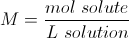Since we have 1mol of protons and 1L of solution, the molarity of protons is simply 1M in the initial solution (1mol/1L). We can plug this into the pH equation and solve.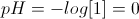2) After the dilution with water, the concentration of protons will change. Since the volume of the solution has doubled, the concentration of protons has been halved.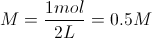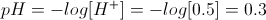* Key points to remember!

1) The lower the pH, the more acidic the solution. This is why the diluted solution was 0.3 and the original, more acidic solution was 0.

2) pH is a logarithmic scale. As a result, a pH of 2 is ten times more acidic than a pH of 3. This is why halving the concentration DID NOT halve the pH.

### Example Question #12 : P H

What is the pH of a solution if you dilute 10mL of a 0.56M NaOH solution into 100mL of water?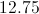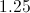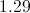There is not enough information to answer this question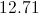Explanation:

We first need to calculate the moles of NaOH in 10mL of a 0.56M solution.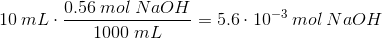Now, in the new volume, the concentration is equal to this same number of moles divided by the new volume. The new volume is the sum of the two solutes together, so the sum of 10mL of solution and 100mL of water.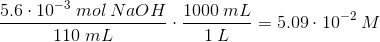Now we need to calculate pH. Since NaOH will dissociate one hydroxide ion for every molecule of NaOH in solution, the concentration of hydroxide is equal to the concentration of NaOH.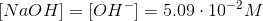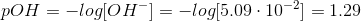Knowing the pH allows us to solve for the pH.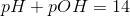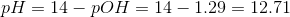### Example Question #11 : Reaction Types

What is the pOH of a solution that has a [H+] = 3.2 X 10-7 mol?

14

-7

6.5

7.5

3.2

7.5

Explanation:

[H+] = 3.2 X 10-7 mol

pH = -log [H+]

= 7 - log 3.2

= 7 -.505

=6.495

pOH = 14 - pH

= 7.5

### Example Question #12 : P H

What is the range of possible hydrogen ion concentrations in basic solution?

10–13 to 10–7.001

7

7 to 14

2 to 3

10–1 to 10–6.999